# HL Triangle

## What are HL Triangles

HL means ‘Hypotenuse-Leg’. HL triangles are right triangles where hypotenuse and one of the other two legs are known. Shown below is a HL triangle, △ABC, where AC is the hypotenuse and AB is one of the other two legs.

## HL Triangle Congruence Theorem

Unlike other congruency postulates, where three quantities are tested, in HL theorem two sides of a right triangle are considered.

### Proving Triangles Congruent using the Hypotenuse-Leg Theorem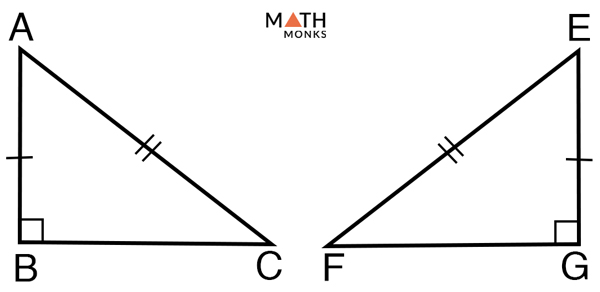Prove HL Triangle Congruence Theorem

To prove:

ΔABC ≅ ΔEGF

Proof:

Given: ΔABC and ΔEGF are right triangles where AB ≅ EG, AC ≅ EF
By Pythagorean Theorem,
In ΔABC,
AC2 = AB2 + BC2 ……. (1), and
In ΔPQR,
EF2 = EG2 + GF2 ……. (2)
Since, AC ≅ EF, from (1) and (2) we can write,
AB2 + BC2 = EG2 + GF2 ……. (3)
Again, AB ≅ EG
Substituting in (3) we get,
EG2 + BC2 = EG2 + GF2
BC2 = GF2
Thus, ΔABC ≅ ΔEGF
Hence Proved

Let us take some examples to understand the concept.

## Solved Examples and Practice Proof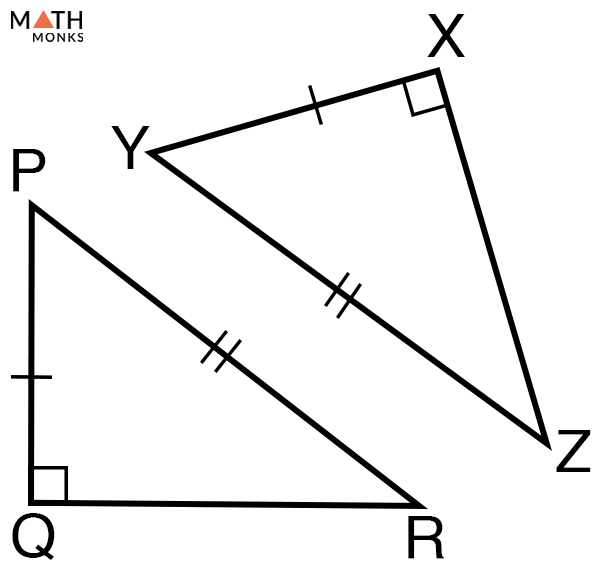Prove whether ∆PQR and ∆YXZ are congruent.

Solution:

Given that ∆PQR and ∆YXZ are right triangles, where
PR ≅ YZ (equal hypotenuse)
PQ ≅ YX (equal leg)
Thus, by ‘Hypotenuse-Leg’ theorem, ∆PQR ≅ ∆YXZ
Hence proved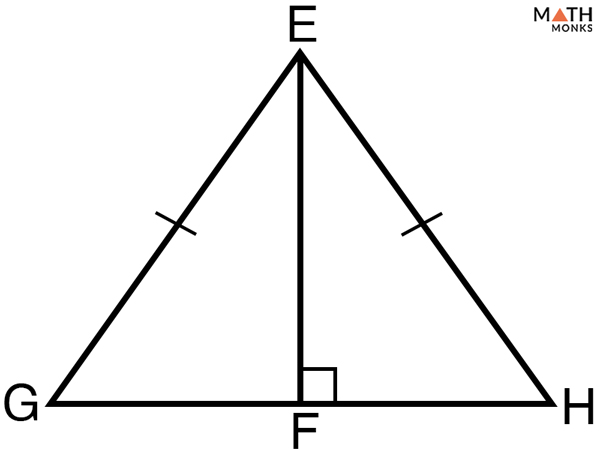Prove that in the given figure, ∆EGF ≅ ∆EHF. Given, EF ⊥ GH and EG ≅ EH.

Solution:

Given that EF ⊥ GH and EG ≅ EH
Thus,
∆EGF and ∆EHF are right triangles as ∠EFG = ∠EFH = 90°
EG ≅ EH (equal hypotenuse)
EF ≅ EF (common leg)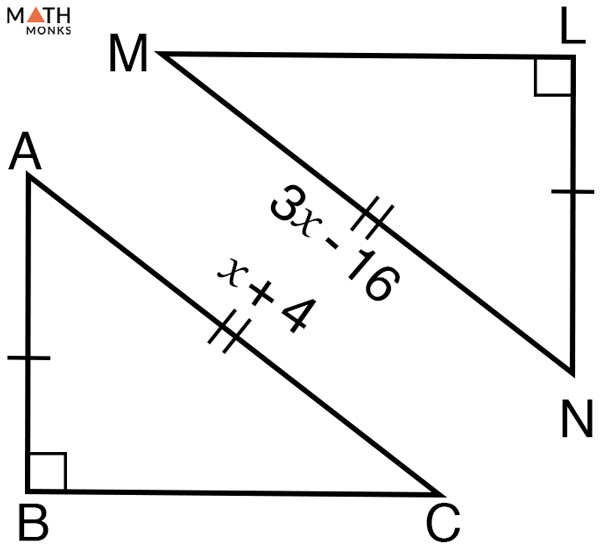Find the value of x in the given pairs of congruent triangles.

Solution:

Given that ∆ABC ≅ ∆NLM
Thus,
x + 4 = 3x – 16
=> x – 3x = – 16 – 4
=> -2x = -20
=> x = 10
Thus, the value of x = 10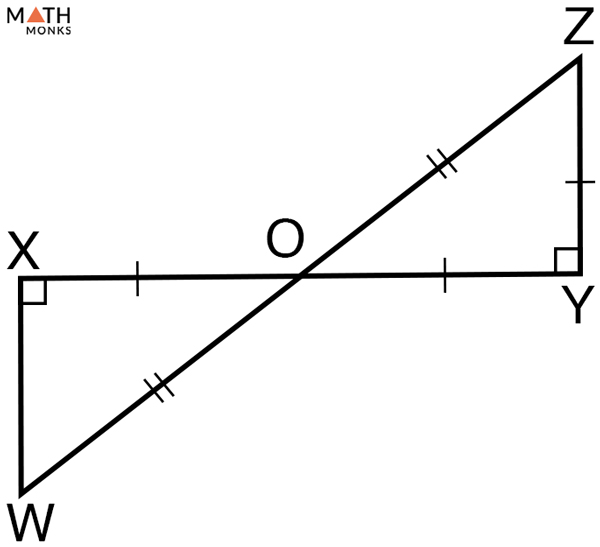Show that ∆OXW and ∆OYZ are congruent. Given that ∠X = ∠Y = 90° and O is the midpoint of XY and WZ.

Solution:

Given that ∆OXW and ∆OYZ are right triangles
∠X = ∠Y = 90°
XO ≅ YO (equal leg)
WO ≅ ZO (equal hypotenuse)
Thus, by the ‘Hypotenuse-Leg’ theorem, ∆OXW ≅ ∆OYZ
Hence proved